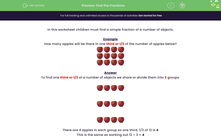# Find Simple Fractions of a Number of Objects

In this worksheet, students will find simple fractions of a number of objects.Key stage:  KS 1

Curriculum topic:   Number: Fractions

Curriculum subtopic:   Recognise Fractions

Popular topics:   Fractions worksheets

Difficulty level:#### Worksheet Overview

In this activity, you will need to find a simple fraction of a number of objects.

Example

How many apples will be there in one third or 1/3 of the number of apples below?To find one third or 1/3 of a number of objects we share or divide them into 3 equal groups.There are 4 apples in each group so one third or 1/3 of 12 is 4

This is the same as working out 12 ÷ 3 = 4

To find one half or 1/2 of a number, we divide by 2.

Can you find 1/2 of these bananas?Share the bananas into 2 equal groups.There are 7 bananas in each group, so 14 ÷ 2 = 7

To find one quarter or 1/4 of a number, we divide by 4.

Can you find 1/4 of these cakes?There are 20 cakes. If we share them into 4 equal groups, there will be 5 in each group: 20 ÷  4 = 5

So 1/4 of 20 is 5.

Are you ready to have a go at some questions now?

### What is EdPlace?

We're your National Curriculum aligned online education content provider helping each child succeed in English, maths and science from year 1 to GCSE. With an EdPlace account you’ll be able to track and measure progress, helping each child achieve their best. We build confidence and attainment by personalising each child’s learning at a level that suits them.

Get started